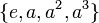# Subgroup structure of cyclic group:Z4

## ContentsAutomorphism class of subgroups List of subgroups (power notation, generator$a$) List of subgroups ($\mathbb{Z}/4\mathbb{Z}$ notation) Isomorphism class Order of subgroups Index of subgroups Number of conjugacy classes(=1 iff automorph-conjugate subgroup) Size of each conjugacy class(=1 iff normal subgroup) Total number of subgroups(=1 iff characteristic subgroup) Isomorphism class of quotient (if exists) Subnormal depth Nilpotency class
trivial subgroup$\{ e \}$$\{ 0 \}$ trivial group 1 4 1 1 1 cyclic group:Z4 1 0
Z2 in Z4$\{ e, a^2 \}$$\{ 0, 2 \}$ cyclic group:Z2 2 2 1 1 1 cyclic group:Z2 1 1
whole group$\{ e, a, a^2, a^3 \}$$\{ 0,1,2,3 \}$ cyclic group:Z4 4 1 1 1 1 trivial group 1 1